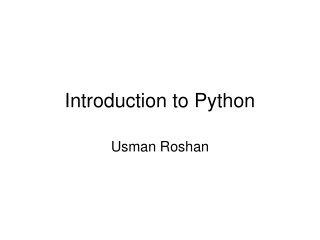DownloadDownload PresentationIntroduction to Python

# Introduction to Python

Télécharger la présentation## Introduction to Python

- - - - - - - - - - - - - - - - - - - - - - - - - - - E N D - - - - - - - - - - - - - - - - - - - - - - - - - - -
##### Presentation Transcript

1. Introduction to Python Usman Roshan

2. Python • Interpreter language: the Python interpreter executes each statement one at a time. • No compilation or linking is required. • Python programs are called scripts which are simple text files. To execute a script we pass it through the interpreter.

3. Data types • Basic data types • Integer • Float • String • Boolean (true or false) • Collections • Lists • Dictionaries (hash tables) • Sets • And more…

4. Expressions • Numeric operators such as +, -, *, /, ** • Logical operators such as and and or • String operators such as in, +, [], [:] • String and numeric functions such as len, abs, max, and min.

5. Assignment • name = value • Examples: • sequence = “ACCCATA” • x = 5 • y = x + 2

6. Lists • Ordered collection of basic types • Examples: • list1 = [1, 2, 3] • list2 = [4, 5] • Operations on lists • Concatenate: list3 = list1 + list2 • Access nth element: list1[n]

7. Lists • Time to lookup, insert, and delete • We care about the worst case runtime for now • We will write runtimes as a function of the input size • The worst case runtime to lookup an item is the length of the list. But the time to lookup the ithitem is actually constant. If we say print(l[i]) where l is a list then this takes constant time. • The worst case runtime to insert an item into the list is the length of the list • The worst case runtime to delete an item from the list is the length of the list

8. Dictionaries • Dictionaries are like arrays, except that they are indexed by user defined keys instead of non-negative integers. • They are also called hash-tables • Example • h={‘A’:’T’, ‘C’:’G’} • Operations on dictionaries • Lookup: h[key]

9. Hash-tables • Time to lookup, insert, and delete • We will write runtimes as a function of the input size • The expected case runtime to lookup an item is constant • The expected case runtime to insert an item into the list is constant • The expected case runtime to delete an item from the list is constant

10. Input and Output • Input and output to files is done by creating a file object open(path, mode) • Example: f = open(“myfile.txt”,”r”) f.readline()

11. Control structures • Conditional statements if expression : statements1 else: statements2 • Example max = 0 if(x > y): max = x else: max = y

12. Iteration • for count in range(start,stop,step): statements • for item in collection: do something with item

13. Basic algorithmic techniques • In mainstream CS one is taught: • Dynamic programming • Greedy algorithms • Divide-and-conquer • In data science and machine learning we use coordinate and gradient descent extensively • What is coordinate and gradient descent?

14. Runtime analysis • Theoretical analysis: How long does it take for an algorithm to finish as a function of input size? • Real analysis: runtime in seconds depending upon machine